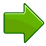# DIFFERENT TYPES OF TRIANGLES AND THERE DEFINATIONS

this is the right answer .....• 1

equilatereal triangle =A triangle having all sides equal

isosceles  triangle =  A triangle having  two sides equal

scalene  triangle  = a triangle having  all sides are of different lengths

• 0

Right angled triangle=  A triangle having a right angle

• -1

hello  gurparteet

your spelling of equilatereal triangle is not correct

it is equilateral triangle

• 0

yep..........!!

Thank  u...

Usually i type fast and sometome  bymistake it happens

• -1
Different types of triangles are -:

equilatereal triangle =A triangle having all sides equal

isosceles? triangle =? A triangle having? two sides equal

scalene? triangle? =?a triangle having? all sides are of different lengths?
• -1

# TrianglesA triangle has three sides and three anglesThe three angles always add to 180°

## Equilateral, Isosceles and Scalene

There are three special names given to triangles that tell how many sides (or angles) are equal.

There can be 32 or no equal sides/angles:### Equilateral Triangle

Three equal sides
Three equal angles, always 60°### Isosceles Triangle

Two equal sides
Two equal anglesNo equal sides
No equal angles

## What Type of Angle?

Triangles can also have names that tell you what type of angle is inside:### Acute Triangle

All angles are less than 90°### Right Triangle

Has a right angle (90°)### Obtuse Triangle

Has an angle more than 90°

## Combining the Names

Sometimes a triangle will have two names, for example:### Right Isosceles Triangle

Has a right angle (90°), and also two equal angles

Can you guess what the equal angles are?

## Play With It ...

Try dragging the points around and make different triangles:

You might also like to play with the Interactive Triangle.

## Perimeter

The perimeter is the distance around the edge of the triangle: just add up the three sides:

## AreaThe area is half of the base times height.

• "b" is the distance along the base
• "h" is the height (measured at right angles to the base)

Area = ½ × b × h

The formula works for all triangles.

Note: a simpler way of writing the formula is bh/2

### Example: What is the area of this triangle?(Note: 12 is the height, not the length of the left-hand side)

Height = h = 12

Base = b = 20

Area = ½ × b × h = ½ × 20 × 12 = 120

The base can be any side, Just be sure the "height" is measured at right angles to the "base":

(Note: You can also calculate the area from the lengths of all three sides using Heron's Formula.)

### Why is the Area "Half of bh"?

Imagine you "doubled" the triangle (flip it around one of the upper edges) to make a square-like shape (a parallelogram) which can be changed to a simple rectangle:THEN the whole area is bh, which is for both triangles, so just one is ½ × bh.

• 2
any-more-doubts?
• -1
Every triangle have 3angles 3vertices and 3edges
• -1
What mining of bando
• -2
the three types are:
>scalene triangle- three sides are unequal
>isosceles triangle-two sides are equal
>equilateral triangle-all sides are equal
• 0
A triangle's type depends on the length of its sides and the size of its angles (corners). There are three types of triangle based on the length of the sides: equilateral, isosceles, and scalene....
• 0
There are three types of angles
Scalene triangle - A triangle which does not has any of its side equal.
Iscosceles triangle - A triangle which has only two of its sides equal.
Equilateral triangle - A triangle that has all its sides equal is known as an equilateral triangle.
• 0
There are two types of triangles:
1.Triangles based on sides
i)Equilateral triangle-All sides are equal.
ii)Isosceles triangle-Any two sides are equal.
iii)Scalene triangle-All sides are of different length.
2.Triangles based on angles
i)Acute angled triangle-All angles are less than 90degrees.
ii)Right angled triangle-One angle measures 90degrees.
iii)Obtuse angled triangle- One angle measure more than 90degrees.
• 0• 0
How many types???
• 0
there are three triangles
• -1
scalene- NONE OF THE SIDES ARE EQUAL
isosceles- ONLY 2 SIDES ARE EQUAL
equilateral- ALL 3 SIDES ARE EQUAL
• 2
equilateral triangle =A triangle having all sides equal isosceles triangle = A triangle having two sides equal scalene triangle = a triangle having all sides are of different lengths Hope it helps😘
• 2
Scalene triangle is a triangle where all the 3 line segments are in different measures
Isosceles triangle is a triangle where 2 line segments are of the same measure .
Equilateral triangle is a triangle where all the 3 line segments are of the same length .
• 0• 0• 0
Can any one please send the algebric set33.
• 0
scalene triangle isosceles triangle and equiletreal triangle

• 0
ijlhlblbj`jbl
• 1
IDk.............
• 0
What are you looking for?